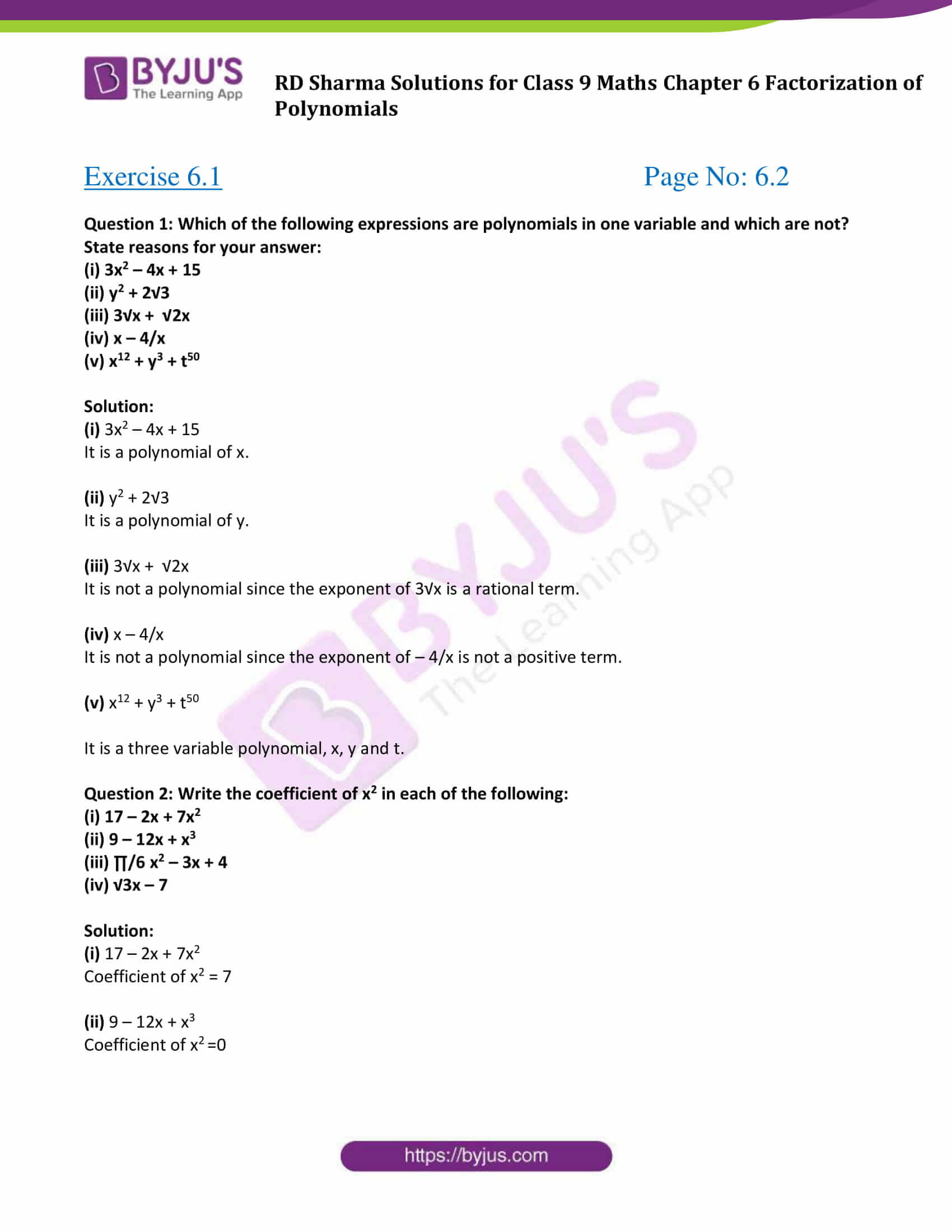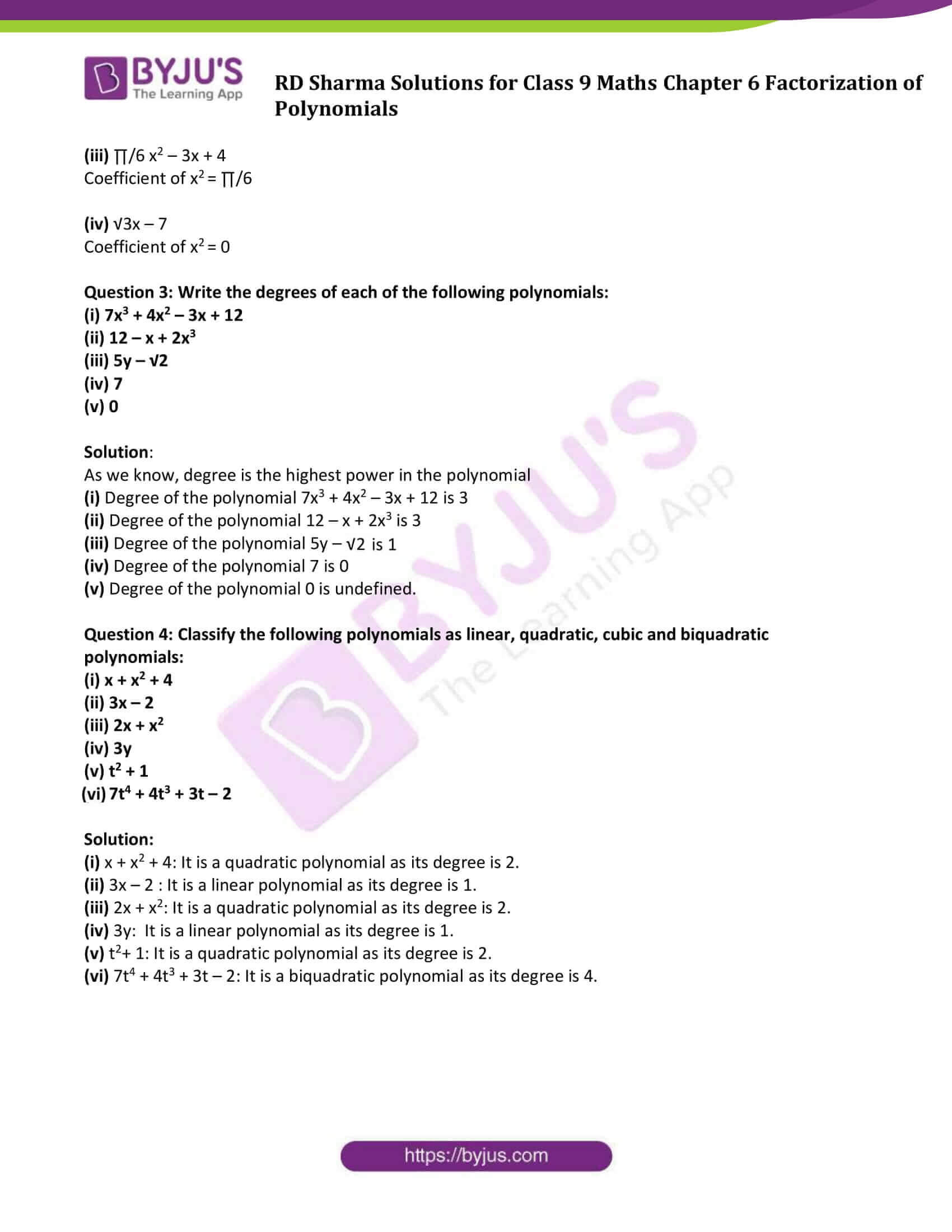# RD Sharma Solutions for Class 9 Maths Chapter 6 Factorization of Polynomials Exercise 6.1

RD Sharma Class 9 Mathematics Chapter 6 Exercise 6.1 Factorization of Polynomials is provided here. Sometimes students get confused between degree and coefficient of a polynomial. Let us have a look at the definition of a polynomial and some of its terms.

A polynomial is an expression in which a combination of constants and variables.

The exponent of the highest degree term in a polynomial is known as its degree whereas the coefficient of the leading term is called the leading coefficient.

In this exercise, students will be dealt with a polynomial in one variable only.

For more details, students can practice RD Sharma Solutions Class 9 chapter 6.

## Download PDF of RD Sharma Solutions for Class 9 Maths Chapter 6 Factorization of Polynomials Exercise 6.1### Access Answers to Maths RD Sharma Solutions for Class 9 Chapter 6 Factorization of Polynomials Exercise 6.1 Page number 6.2

#### Exercise 6.1 Page No: 6.2

Question 1: Which of the following expressions are polynomials in one variable and which are not? State reasons for your answer:

(i) 3x2 – 4x + 15

(ii) y2 + 2√3

(iii) 3√x + √2x

(iv) x – 4/x

(v) x12 + y3 + t50

Solution:

(i) 3x2 – 4x + 15

It is a polynomial of x.

(ii) y2 + 2√3

It is a polynomial of y.

(iii) 3√x + √2x

It is not a polynomial since the exponent of 3√x is a rational term.

(iv) x – 4/x

It is not a polynomial since the exponent of – 4/x is not a positive term.

(v) x12 + y3 + t50

It is a three variable polynomial, x, y and t.

Question 2: Write the coefficient of x2 in each of the following:

(i) 17 – 2x + 7x2

(ii) 9 – 12x + x3

(iii) ∏/6 x2 – 3x + 4

(iv) √3x – 7

Solution:

(i) 17 – 2x + 7x2

Coefficient of x2 = 7

(ii) 9 – 12x + x3

Coefficient of x2 =0

(iii) ∏/6 x2 – 3x + 4

Coefficient of x2 = ∏/6

(iv) √3x – 7

Coefficient of x2 = 0

Question 3: Write the degrees of each of the following polynomials:

(i) 7x3 + 4x2 – 3x + 12

(ii) 12 – x + 2x3

(iii) 5y – √2

(iv) 7

(v) 0

Solution:

As we know, degree is the highest power in the polynomial

(i) Degree of the polynomial 7x3 + 4x2 – 3x + 12 is 3

(ii) Degree of the polynomial 12 – x + 2x3 is 3

(iii) Degree of the polynomial 5y – √2 is 1

(iv) Degree of the polynomial 7 is 0

(v) Degree of the polynomial 0 is undefined.

Question 4: Classify the following polynomials as linear, quadratic, cubic and biquadratic polynomials:

(i) x + x2 + 4

(ii) 3x – 2

(iii) 2x + x2

(iv) 3y

(v) t2 + 1

(vi) 7t4 + 4t3 + 3t – 2

Solution:

(i) x + x2 + 4: It is a quadratic polynomial as its degree is 2.

(ii) 3x – 2 : It is a linear polynomial as its degree is 1.

(iii) 2x + x2: It is a quadratic polynomial as its degree is 2.

(iv) 3y: It is a linear polynomial as its degree is 1.

(v) t2+ 1: It is a quadratic polynomial as its degree is 2.

(vi) 7t4 + 4t3 + 3t – 2: It is a biquadratic polynomial as its degree is 4.

## RD Sharma Solutions for Class 9 Maths Chapter 6 Factorization of Polynomials Exercise 6.1

RD Sharma Solutions Class 9 Maths Chapter 6 Factorization of Polynomials Exercise 6.1 is based on the following topics and subtopics:

• Factorization of Polynomials introduction
• Terms and coefficients
• Degree of a polynomial
• Constant polynomial
• Linear polynomial